# CASIO

User´s Guide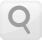## Configuring the Calculator Setup

Pressing the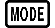key more than once displays additional setup screens.
Underlined ( ___ ) settings are initial defaults.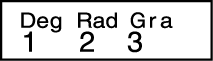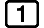Deg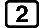Rad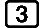Gra
Specifies degrees, radians or grads as the angle unit for value input and calculation result display.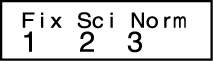FixSciNorm
Specifies the number of digits for display of a calculation result.

Fix: The value you specify (from 0 to 9) controls the number of decimal places for displayed calculation results. Calculation results are rounded off to the specified digit before being displayed.

Example: 100 ÷ 7 = 14.286 (Fix 3)

Example: 100 ÷ 7 = 14.29 (Fix 2)

Sci: The value you specify (from 1 to 10) controls the number of significant digits for displayed calculation results. Calculation results are rounded off to the specified digit before being displayed.

Example: 1 ÷ 7 = 1.4286 × 10-1 (Sci 5)

Example: 1 ÷ 7 = 1.428571429 × 10-1 (Sci 0)

Norm: Selecting one of the two available settings (Norm 1, Norm 2) determines the range in which results will be displayed in exponential format. Outside the specified range, results are displayed using non-exponential format.

Norm 1: 10-2 > |x|, |x| ≧ 1010
Norm 2: 10-9 > |x|, |x| ≧ 1010

Example: 1 ÷ 200 = 5 × 10-3 (Norm 1)

Example: 1 ÷ 200 = 0.005 (Norm 2)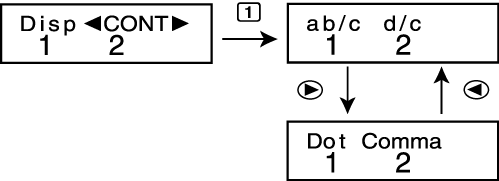ab/cd/c
Specifies either mixed fraction (ab/c) or improper fraction (d/c) for display of fractions in calculation results.DotComma
Specifies whether to display a dot or a comma for the calculation result decimal point. A dot is always displayed during input.
Dot: Period decimal point, comma separator
Comma: Comma decimal point, period separator

### Note

To close the setup menu without selecting anything, press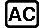.

## Initializing the Calculation Mode and Other Settings

Performing the following procedure initializes the calculation mode and other setup settings as shown below.

fx-82MS/fx-85MS/fx-300MS/fx-350MS: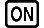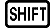(CLR)(Mode)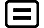fx-220 PLUS: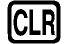(Mode)This setting: Is initialized to this: COMP Deg Norm 1 a b/c Dot

To cancel initialization without doing anything, press(Cancel) instead of.